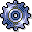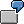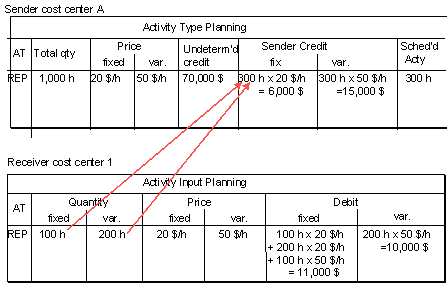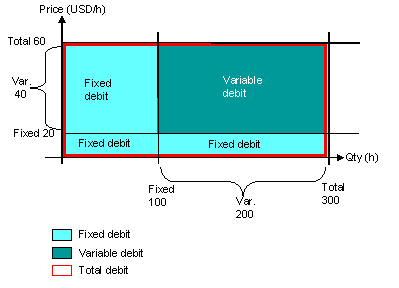Activity-Dependent Activity Input PlanningUse

With activity-dependent activity input planning you can plan secondary costs dependent on an activity type in the receiver. You plan the input of activity type Y of sender cost center A for activity type X of receiver cost center 1. Activity type Y of sender cost center A is required to produce activity type X on receiver cost center 1.

Features

The SAP System posts the planned debit of the receiver cost center under a secondary cost element for internal activity allocation. If you have not manually planned the prices during activity type planning, the SAP System calculates the debit for the receiver cost center using the iteratively calculated price.

The system:

• Credits the sender cost center by the amount of the plan activity quantity multiplied by the price (manual or iterative). This amount is split into a total amount and a fixed amount.
• Debits the receiver cost center with the product of the activity quantity received multiplied by the price. The example below illustrates how costs are split into fixed and total portions.Receiver cost center 1 takes 300 hours of repair activity (REP) from sender cost center A. The following plan data results:The debit to the receiver is calculated as follows:

• Total debit

The total debit to the receiver for an activity type equals the credit to the sender for this activity type, that is, the total activity quantity input X overall price.

• Fixed debits

The fixed debit to the receiver for an activity type is derived from the fixed activity input. The fixed activity input is incurred irrespective of the activity to be produced on the receiver cost center. The fixed debit is composed of two elements.

1. The total activity quantity input multiplied by the fixed price,
All costs that were fixed on the sender cost center remain fixed on the receiver cost center.
2. The fixed activity quantity input multiplied by the variable portion of the price.

The fixed debit is comprised of the total costs of the activity input without the variable costs (variable quantity of activity input multiplied by the variable price).

The credit to the sender is calculated as follows:

• Fixed credit

Total quantity X fixed price

• Variable credit

Total quantity X variable price